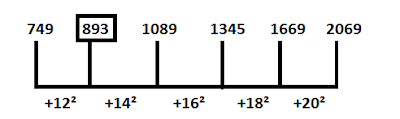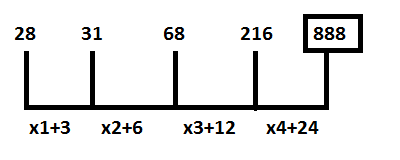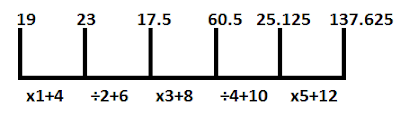# Number Series Questions for IPPB Exam

## Set I

Directions (1-5): In each of the following number series one number is wrong, Find out the wrong number.

#### Ques 1)

749, 890, 1089, 1345, 1669, 2069
1. 1669
2. 749
3. 890
4. 2069
5. 1089
3; The series isHence, there is should be 893 in place of 890.

#### Ques 2)

39, 235, 1647, 13289, 118615
1. 235
2. 39
3. 118615
4. 1647
5. 13289

5; The series isHence, there should be 13179 in place of 13289

#### Ques 3)

53, 217, 1943, 31103, 77555
1. 77555
2. 217
3. 1943
4. 53
5. 31103

1; The series isHence, there should be 7775555 in place of 77555.

#### Ques 4)

2394, 798, 3192, 688.4, 3830.4, 547.2
1. 3830.4
2. 547.2
3. 688.4
4. 798
5. 3192

3; The series isHence, there should be 638.4 in place of 688.4

#### Ques 5)

28, 31, 68, 216, 896
1. 896
2. 28
3. 31
4. 216
5. 68

1; The series isHence, there should be 888 in place of 896.

## Set - II

Directions (1-5): What is the value should come in place of question mark (?) in the following number series?

#### Ques 1)

48, ?, 94, 123, 156, 193
1. 74
2. 64
3. 65
4. 69
5. 76

4; The series is:
2² + (11 x 4) = 48
3² + (12 x 5) = 69
4² + (13 x 6) = 94
5² + (4 x 7) = 123
6² + (5 x 8) = 156
7² + (16 x 9) = 193

#### Ques 2)

37, ?, 65, 82, 101, 122
1. 50
2. 55
3. 45
4. 42
5. 48

1; The series is :
8 x 3 + 13 = 37
9 x 4 + 14 = 50
10 x 5 + 15 = 65
11 x 6 + 16 = 82
12 x 7 + 17 x 101
13 x 8 + 18 = 122

#### Ques 3)

8, 48, 32, 192, ?, 1056
1. 181
2. 176
3. 167
4. 185
5. 171

2; The series is#### Ques 4)

14, 28, 46, ?, 94, 124
1. 64
2. 76
3. 82
4. 72
5. 68

5; The series is :
4 x 2 + 6 = 14
5 x 4 + 8 = 28
6 x 6 + 10 = 46
7 x 8 + 12 = 68
8 x 10 + 14 = 94
9 x 12 + 16 = 124

#### Ques 5)

19, 23, 17.5, 60.5, ?, 137.625
1. 21.125
2. 24.125
3. 20.125
4. 30.125
5. 25.125

5; The series is#### What's trending in BankExamsToday

Smart Prep Kit for Banking Exams by Ramandeep Singh - Download here